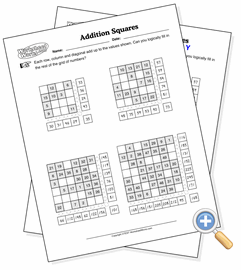# Addition SquaresDevelop problem solving strategies with sums of integers

This exercise is a favorite of teachers for helping students learn logical problem-solving skills, and works great in teams of two. The addition square has different sums in each row, column and diagonal (as opposed to magic squares, which have the same sum). You can configure this worksheet to either ask for the sums, which is relatively straightforward, or provide the sums and ask for the grid to be completed. In the second case, the puzzle will always provide just enough filled squares to allow the student to deduce all of the missing squares.